Next: MATHEMATICAL ANALYSIS Up: Lumley: On the validity Previous: Introduction

# VALIDITY CONDITION RESULTS

In this section I will state the validity condition results of the mathematical analysis that follows in the main body of the paper. A valid acoustic wave equation governing fluid particle motion for a pressure wavefield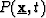is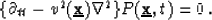(1)

1. It is perfectly rigorous to use (1) in a linear isotropic non-viscous fluid with a constant mass density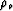and a spatially variable bulk modulus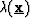such that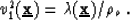(2)

2. In reasonable'' background models wherevaries smoothly with respect to pressure wavelength, 1 can be extended to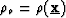such that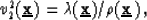(3)

when the following validity condition is satisfied: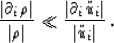(4)

Condition (4) is invalid near regions of discontinuities in(e.g., reflecting boundaries), but is valid in regions of smooth-varying spatial properties (e.g., a smoothed migration model).Next: MATHEMATICAL ANALYSIS Up: Lumley: On the validity Previous: Introduction
Stanford Exploration Project
11/18/1997